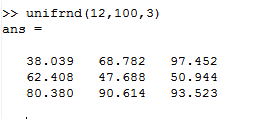# Matrix in Scala

I am new to Scala with background of Matlab. I want some mechanism to Return a matrix of random samples from the uniform distribution.In Matlab this could be done with unifrnd. How to perform same task in Scala.
Matlab code image is here with 12 as lower bound and 100 is upper bound and 3 is size of matrix.If you use a numerical computing library like `scalanlp/breeze`, it will probably have this functionality. Example: https://github.com/scalanlp/breeze/blob/master/math/src/main/scala/breeze/linalg/Matrix.scala#L242-L244

1 Like

If you are happy with an Array[Array[Double]] for your matrix then you can do it with a call to fill.

``````scala> Array.fill(3, 3)(88*math.random+12)
res1: Array[Array[Double]] = Array(Array(61.522605977730485, 57.49302550510446, 60.41657496484339), Array(26.91129563629221, 70.50596757746433, 51.77716898846029), Array(96.69968605646909, 70.64093890889811, 21.969996915976523))
``````

However, if you really want Matrix operations then Breeze (mentioned by virus_dave) or ScalaLab (https://github.com/sterglee/scalalab) might be better choices.

1 Like

Thanks ,it’s done.